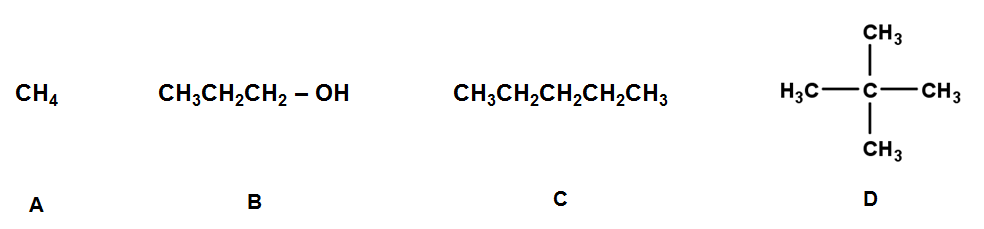# Problem: Rank the following compounds in order of increasing vapor pressure.(a) A &lt; B &lt; C &lt; D(b) D &lt; C &lt; B &lt; A(c) A &lt; D &lt; C &lt; B(d) B &lt; C &lt; D &lt; A(e) A &lt; C ≈ D &lt; B

###### FREE Expert Solution

We are being asked to rank the given compounds in order of increasing vapor pressure.

Vapor pressure

▪ is the pressure exerted by a vapor in equilibrium with its condensed phase
▪ highly influenced by intermolecular forces between molecules

Intermolecular Forces (IMF) are the attractive forces between 2 molecules.

Ion-dipole

strongest IMF
▪ deals with the attraction between an ion and a polar compound.

Hydrogen Bonding

93% (4 ratings)###### Problem Details

Rank the following compounds in order of increasing vapor pressure.(a) A < B < C < D

(b) D < C < B < A

(c) A < D < C < B

(d) B < C < D < A

(e) A < C ≈ D < B